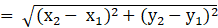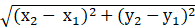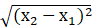# Straight lines Chapter 10 Class 11 Maths NCERT Textbook With Solutions PDF

NCERT Solutions for Class 11 Maths Chapter 10‘ PDF Quick download link is given at the bottom of this article. You can see the PDF demo, size of the PDF, page numbers, and direct download Free PDF of ‘Ncert Class 11 Maths Chapter 10 Exercise Solution’ using the download button.

### Chapter 10: Straight lines

#### 10.1 Introduction

We are familiar with two-dimensional coordinate geometry from earlier classes. Mainly, it is a combination of algebra and geometry.

A systematic study of geometry by the use of algebra was first carried out by celebrated French philosopher and mathematician René Descartes, in his book ‘La Géométry, published in 1637.

This book introduced the notion of the equation of a curve and related analytical methods into the study of geometry.

The resulting combination of analysis and geometry is referred now to as analytical geometry.

In the earlier classes, we initiated the study of coordinate geometry, where we studied coordinate axes, coordinate planes, plotting of points in a plane, the distance between two points, section formulae, etc. All these concepts are the basics of coordinate geometry.

#### 10.2.1 Slope of a line when coordinates of any two points on the line are given

We know that a line is completely determined when we are given two points on it. Hence, we proceed to find the slope of a line in terms of the coordinates of two points on the line.

#### 10.3 Various Forms of the Equation of a Line

We know that every line in a plane contains infinitely many points on it. This relationship between lines and points leads us to find the solution to the following problem:

How can we say that a given point lies on the given line? Its answer may be that for a given line we should have a definite condition on the points lying on the line.

Suppose P (x, y) is an arbitrary point in the XY-plane and L is the given line. For the equation of L, we wish to construct a statement or condition for the point P that is true, when P is on L, otherwise false.

Of course, the statement is merely an algebraic equation involving the variables x and y. Now, we will discuss the equation of a line under different conditions.

### NCERT Solutions Class 11 Maths Chapter 10 Straight lines

1. Draw a quadrilateral in the Cartesian plane, whose vertices are (– 4, 5), (0, 7), (5, – 5), and (– 4, –2). Also, find its area.

Solution:

Let ABCD be the given quadrilateral with vertices A (-4,5), B (0,7), C (5.-5), and D (-4,-2).

Now let us plot the points on the Cartesian plane by joining the points AB, BC, CD, AD which gives us the required quadrilateral.

To find the area, draw diagonal AC

So, area (ABCD) = area (∆ABC) + area (∆ADC)

Then, area of triangle with vertices (x1,y1) , (x2, y2) and (x3,y3) is

Are of ∆ ABC = ½ [x1 (y2 – y3) + x2 (y3 – y1) + x3 (y1 – y2)]

= ½ [-4 (7 + 5) + 0 (-5 – 5) + 5 (5 – 7)] unit2

= ½ [-4 (12) + 5 (-2)] unit2

= ½ (58) unit2

= 29 unit2

Are of ∆ ACD = ½ [x1 (y2 – y3) + x2 (y3 – y1) + x3 (y1 – y2)]

= ½ [-4 (-5 + 2) + 5 (-2 – 5) + (-4) (5 – (-5))] unit2

= ½ [-4 (-3) + 5 (-7) – 4 (10)] unit2

= ½ (-63) unit2

= -63/2 unit2

Since area cannot be negative area ∆ ACD = 63/2 unit2

Area (ABCD) = 29 + 63/2

= 121/2 unit2

2. The base of an equilateral triangle with side 2a lies along the y-axis such that the mid-point of the base is at the origin. Find vertices of the triangle.

Solution:

Let us consider ABC to be the given equilateral triangle with side 2a.

Where, AB = BC = AC = 2a

In the above figure, by assuming that the base BC lies on the x-axis such that the mid-point of BC is at the origin i.e. BO = OC = a, where O is the origin.

The coordinates of point C are (0, a) and that of B is (0, -a)

Since the line joining a vertex of an equilateral ∆ with the mid-point of its opposite side is perpendicular.

So, vertex A lies on the y –axis

By applying Pythagoras’ theorem

(AC)2 = OA2 + OC2

(2a)2= a2 + OC2

4a2 – a2 = OC2

3a= OC2

OC =√3a

Co-ordinates of point C = ± √3a, 0

∴ The vertices of the given equilateral triangle are (0, a), (0, -a), (√3a, 0)

Or (0, a), (0, -a) and (-√3a, 0)

3. Find the distance between P (x1, y1) and Q (x2, y2) when: (i) PQ is parallel to the y-axis, (ii) PQ is parallel to the x-axis.

Solution:

Given:

Points P (x1, y1) and Q(x2, y2)

(i) When PQ is parallel to y-axis then x1 = x2

So, the distance between P and Q is given by= |y2 – y1|

(ii) When PQ is parallel to the x-axis then y1 = y2

So, the distance between P and Q is given by == |x2 – x1|

4. Find a point on the x-axis, which is equidistant from the points (7, 6) and (3, 4).

Solution:

Let us consider (a, 0) to be the point on the x-axis that is equidistant from the point (7, 6) and (3, 4).

So,

Now, let us square on both the sides we get,

a2 – 14a + 85 = a2 – 6a + 25

-8a = -60

a = 60/8

= 15/2

∴ The required point is (15/2, 0)

5. Find the slope of a line, which passes through the origin, and the mid-point of the line segment joining the points P (0, – 4) and B (8, 0).

Solution:

The co-ordinates of mid-point of the line segment joining the points P (0, – 4) and B (8, 0) are (0+8)/2, (-4+0)/2 = (4, -2)

The slope ‘m’ of the line non-vertical line passing through the point (x1, y1) and

(x2, y2) is given by m = (y2 – y1)/(x2 – x1) where, x ≠ x1

The slope of the line passing through (0, 0) and (4, -2) is (-2-0)/(4-0) = -1/2

∴ The required slope is -1/2.